# Greater antidote

This item is of drink class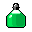You see a greater antidote, to cure you of poison and protect you for a long while.
Attributes
IMMUNIZATION
poisoned
AMOUNT
800
FREQUENCY
1
REGEN
0
Dropped by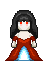vampire bride
Drops between 1 and 2.
Probability: 50%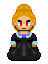kalavan wisewoman
Drops exactly 1.
Probability: 30%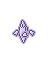ice elemental
Drops between 1 and 3.
Probability: 10%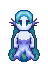water elemental
Drops between 1 and 3.
Probability: 10%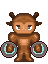earth elemental
Drops between 1 and 3.
Probability: 20%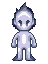air elemental
Drops between 1 and 2.
Probability: 10%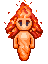fire elemental
Drops between 1 and 3.
Probability: 10%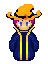imperial high priest
Drops between 1 and 2.
Probability: 2%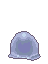twilight slime
Drops exactly 1.
Probability: 100%water demon
Drops between 1 and 2.
Probability: 20%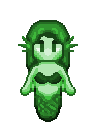emerald mermaid
Drops between 1 and 2.
Probability: 0.1%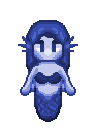sapphire mermaid
Drops between 1 and 2.
Probability: 0.1%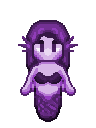amethyst mermaid
Drops between 1 and 2.
Probability: 0.1%